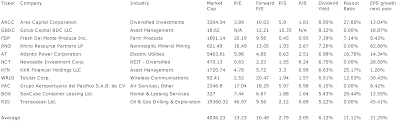## Pages

### 11 High Yield Stocks With Dividend Increasing Potential

High Yield Stocks with Dividend Increasing Potential by Dividend Yield - Stock, Capital, Investment. Here is a current sheet of 11 high yielding dividend stocks (dividend yield of more than 5 percent) that have potential to increase its further dividend. Such stocks have a dividend payout ratio of less than 30 percent as well as positive earnings per share growth for fiscal 2011.

The average price to earnings ratio (P/E ratio) amounts to 13.23 while the forward price to earnings ratio is 10.49. The dividend yield has an average value of 6.12 percent; roughly 11 percent of the income was paid out. Price to book ratio is 2.05 and price to sales ratio 2.79. The expected growth in earnings per share amounts to 21.25 percent.

Here is the table of high yielding stocks with additional dividend increasing potential:High Yields with Dividend Increasing Potential (Click to enlarge)